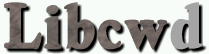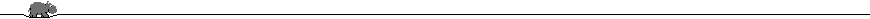The C++ Debugging Support Library By Carlo Wood, ©1999 - 2003.

In this tutorial you will learn how to make memory allocations «invisible» so that they will not show up in the Allocated memory Overview, how to find information about an allocated memory block given an arbitrary pointer pointing inside it and how to write simple memory-leak detection code.

### 7.1 Removing allocations from the Allocated memory Overview

Sometimes a program can allocate a very large number of memory blocks.  Having all of those in the Allocated memory Overview could make it impractically large.  Therefore it is possible to remove items from this list.

In the following example we make one allocation invisible by using the function `make_invisible()`:

Compile as: `g++ -g -DCWDEBUG test7.1.1.cc -lcwd -o invisible`

```
#include "sys.h"
#include "debug.h"
#include <cstdlib>

int main()
{
Debug(libcw_do.on());
Debug(dc::malloc.on());

int* first = new int;
AllocTag2(first, "first");

int* second = new int;
AllocTag2(second, "second");

Debug(list_allocations_on(libcw_do));

Debug(make_invisible(first));

Debug(list_allocations_on(libcw_do));

delete second;
delete first;
}

```

The output of this program is

```
MALLOC  : operator new (size = 4) = 0x55dc6adb8780 [test7.1.1.cc:10]
MALLOC  : operator new (size = 4) = 0x55dc6adb8550 [test7.1.1.cc:13]
MALLOC  : Allocated memory: 72712 bytes in 3 blocks.
0x55dc6adb8550         test7.1.1.cc:13   int; (sz = 4)  second
0x55dc6adb8780         test7.1.1.cc:10   int; (sz = 4)  first
malloc    0x55dc6abcc350          eh_alloc.cc:123  <unknown type>; (sz = 72704)
MALLOC  : Allocated memory: 72708 bytes in 2 blocks.
0x55dc6adb8550         test7.1.1.cc:13   int; (sz = 4)  second
malloc    0x55dc6abcc350          eh_alloc.cc:123  <unknown type>; (sz = 72704)
MALLOC  : delete 0x55dc6adb8550         test7.1.1.cc:13   int; (sz = 4)  second
```

As you can see, the first allocation at line 9 disappeared from the overview after it was made invisible.

Pointer validation at de-allocation is still performed however.  For instance, when we make a mistake when freeing the first `int`:

Compile as: `g++ -g -DCWDEBUG test7.1.2.cc -lcwd -o coredump`

```
#include "sys.h"
#include "debug.h"

int main()
{
Debug(libcw_do.on());
Debug(dc::malloc.on());

int* first = new int;
AllocTag2(first, "first");

int* second = new int;
AllocTag2(second, "second");

Debug(list_allocations_on(libcw_do));

Debug(make_invisible(first));

Debug(list_allocations_on(libcw_do));

delete second;
#pragma clang diagnostic ignored "-Wmismatched-new-delete"
delete [] first;	// Make a deliberate error
}

```

then the output becomes

```
MALLOC  : operator new (size = 4) = 0x55fff75b1950 [test7.1.2.cc:9]
MALLOC  : operator new (size = 4) = 0x55fff75b1720 [test7.1.2.cc:12]
MALLOC  : Allocated memory: 72712 bytes in 3 blocks.
0x55fff75b1720         test7.1.2.cc:12   int; (sz = 4)  second
0x55fff75b1950         test7.1.2.cc:9    int; (sz = 4)  first
malloc    0x55fff73c5350          eh_alloc.cc:123  <unknown type>; (sz = 72704)
MALLOC  : Allocated memory: 72708 bytes in 2 blocks.
0x55fff75b1720         test7.1.2.cc:12   int; (sz = 4)  second
malloc    0x55fff73c5350          eh_alloc.cc:123  <unknown type>; (sz = 72704)
MALLOC  : delete 0x55fff75b1720         test7.1.2.cc:12   int; (sz = 4)  second
COREDUMP: You are `delete[]'-ing a block that was allocated with `new'!  Use `delete' instead.
```

Also the function `test_delete()` still works:

Compile as: `g++ -g -DCWDEBUG test7.1.3.cc -lcwd -o test_delete`

```
#include "sys.h"
#include "debug.h"
#include <cstdlib>

int main()
{
Debug(libcw_do.on());
Debug(dc::malloc.on());
Debug(dc::notice.on());

void* p = std::malloc(3000);

Debug(make_invisible(p));
Debug(list_allocations_on(libcw_do));

#if CWDEBUG_ALLOC
Dout(dc::notice, "test_delete(" << p << ") = " << test_delete(p));
#endif
std::free(p);
#if CWDEBUG_ALLOC
Dout(dc::notice, "test_delete(" << p << ") = " << test_delete(p));
#endif
}

```

results in

```
MALLOC  : malloc(3000) = 0x55650f9bf060 [test7.1.3.cc:11]
MALLOC  : Allocated memory: 72704 bytes in 1 blocks.
malloc    0x55650f7cd350          eh_alloc.cc:123  <unknown type>; (sz = 72704)
NOTICE  : test_delete(0x55650f9bf060) = 0
NOTICE  : test_delete(0x55650f9bf060) = 1
```

However, `find_alloc()`, the function that is explained in the next paragraph, will fail to find an «invisible» block (it will return `NULL`).

### 7.2 Retrieving information about memory allocations

Libcwd allows the developer to generate powerful debugging output; aside from being able to test if a given pointer points to the start of an allocated memory block, using `test_delete()`, it is even possible to find all information about an allocated memory block that is also shown in the Allocated memory Overview when given a pointer pointing anywhere inside an allocated memory block.  For example:

Compile as: `g++ -g -DCWDEBUG test7.2.1.cc -lcwd -o find_alloc`

```
#include "sys.h"
#include "debug.h"

using namespace libcwd;

template<typename T1, typename T2>
struct Foo {
T1 for_me;
T2 for_you;
double d;
};

int main()
{
Debug(libcw_do.on());
Debug(dc::malloc.on());
Debug(dc::notice.on());

Foo<char, int>* f = new Foo<char, int>;
AllocTag(f, "Our test object");

int* p = &f->for_you;	// Pointer that points inside `f'

Dout(dc::notice, "f == " << static_cast<void*>(f));
Dout(dc::notice, "p == " << static_cast<void*>(p));

#if CWDEBUG_ALLOC
alloc_ct const* alloc = find_alloc(p);
Dout(dc::notice,
"p points inside \""
<< alloc->description()
<< "\" starting at "
<< alloc->start()
<< " with size "
<< alloc->size()
<< '.');
Dout(dc::notice,
"This memory block contains a \""
<< alloc->type_info().demangled_name() << "\".");
Dout(dc::notice,
"The allocation type is `"
<< alloc->memblk_type()
<< "' and was allocated at "
<< alloc->location()
<< '.');
#endif
}

```

The output of this program is

```
MALLOC  : operator new (size = 16) = 0x556447e93b70 [test7.2.1.cc:19]
NOTICE  : f == 0x556447e93b70
NOTICE  : p == 0x556447e93b74
NOTICE  : p points inside "Our test object" starting at 0x556447e93b70 with size 16.
NOTICE  : This memory block contains a "Foo<char, int>*".
NOTICE  : The allocation type is `memblk_type_new' and was allocated at test7.2.1.cc:19.
```

Note that the type returned by `alloc->type_info().demangled_name()` is the type of the pointer passed to `AllocTag()`: This string will always end on a `'*'`

The original reason for supporting pointers that point inside a memory block and not just to the start of it, was so that it could be used in base classes of objects derived with multiple inheritance: `find_alloc(this)` will always return the correct memory block, even if there is an offset between `this` and the real start of the allocated block.  The same holds for arrays of objects allocated with `new[]`.

It is also possible to get the values for the number of bytes and blocks allocated in total, as is printed at the top of each Allocated memory Overview.  See the next paragraph.

### 7.3 Memory leak detection

`mem_blocks()` and `mem_size()` (like everything else defined in namespace `libcwd`) can be used to write a very simply memory leak detection system:

Compile as: `g++ -g -DCWDEBUG test7.3.1.cc -lcwd -o total_alloc`

```
#include "sys.h"
#include "debug.h"

using namespace libcwd;

int main()
{
Debug(libcw_do.on());
Debug(dc::malloc.on());

char* memory_leak = new char ;
AllocTag(memory_leak, "memory_leak");

// Debug(make_invisible(memory_leak));

#if CWDEBUG_ALLOC
if (mem_blocks() > 0)
Dout(dc::warning, "There are still " << mem_size() << " bytes allocated!");
else
Dout(dc::malloc, "No memory leaks.");
#endif
}

```

The output of this program is:

```
MALLOC  : operator new[] (size = 300) = 0x5575d5d7cd50 [test7.3.1.cc:11]
WARNING : There are still 73004 bytes allocated!
```

Invisible blocks are not detected!  When you comment out the `Debug(make_invisible(memory_leak));` then the output will read

```MALLOC  : operator new[] (size = 300) = 0x804fc88
MALLOC  : No memory leaks.
```

This allows you to improve the memory leak detection a bit in the case of global objects that allocate memory.  Nevertheless, that is bad coding: you shouldn't define global objects that allocate memory.

Here is an example program anyway:

Compile as: `g++ -g -DCWDEBUG test7.3.2.cc -lcwd -o memleak`

```
#include "sys.h"
#include "debug.h"

class A {
private:
char* dynamic_memory;
public:
A()
{
dynamic_memory = new char ;
AllocTag(dynamic_memory, "A::dynamic_memory");
}
~A()
{
if (dynamic_memory)
delete [] dynamic_memory;
}
};

// Global object that allocates memory (bad programming!)
A a;

int main()
{
Debug(libcw_do.on());
Debug(dc::malloc.on());

#if CWDEBUG_ALLOC
if (libcwd::mem_blocks() > 0)
{
Dout(dc::malloc|dc::warning, "Memory leak");
Debug(list_allocations_on(libcw_do));
}
else
Dout(dc::malloc, "No memory leaks.");
#endif
}

```

results in

```
MALLOC  : Memory leak
MALLOC  : Allocated memory: 73004 bytes in 2 blocks.
new[]     0x55ff811bb840         test7.3.2.cc:10   char; (sz = 300)  A::dynamic_memory
malloc    0x55ff80fef350          eh_alloc.cc:123  <unknown type>; (sz = 72704)
MALLOC  : delete[] 0x55ff811bb840         test7.3.2.cc:10   char; (sz = 300)  A::dynamic_memory
```

simply because `A::dynamic_memory` is not deleted until after `main`.

A simple kludge is to make all memory allocated before `main`, invisible:

Compile as: `g++ -g -DCWDEBUG test7.3.3.cc -lcwd -o memleak2`

```
#include "sys.h"
#include "debug.h"

class A {
private:
char* dynamic_memory;
public:
A()
{
dynamic_memory = new char ;
AllocTag(dynamic_memory, "A::dynamic_memory");
}
~A()
{
if (dynamic_memory)
delete [] dynamic_memory;
}
};

// Global object that allocates memory (bad programming!)
A a;

int main()
{
Debug(make_all_allocations_invisible_except(nullptr));

Debug(libcw_do.on());
Debug(dc::malloc.on());

#if CWDEBUG_ALLOC
if (libcwd::mem_blocks() > 0)
{
Dout(dc::malloc|dc::warning, "Memory leak");
Debug(list_allocations_on(libcw_do));
}
else
Dout(dc::malloc, "No memory leaks.");
#endif
}

```

which will simply output

```
MALLOC  : No memory leaks.
```

Libcwd provides an alternative way to check for memory leaks using so called «markers».  A marker is like a directory, any allocation made after a marker is created is put into that directory.  When a marker is removed and there are still allocation inside it, you will get a warning!  In the following example we allocate a memory block, then set a marker and next allocate two more memory blocks.  The Allocated memory Overview is then printed.

Compile as: `g++ -g -DCWDEBUG test7.3.4.cc -lcwd -o marker`

```
#include "sys.h"
#include "debug.h"

int main()
{
Debug(make_all_allocations_invisible_except(NULL));
Debug(libcw_do.on());
Debug(dc::malloc.on());

int* p1 = new int ;
AllocTag(p1, "p1");

#if CWDEBUG_MARKER
libcwd::marker_ct* marker = new libcwd::marker_ct("A test marker");
#endif

int* p2 = new int ;
AllocTag(p2, "p2");

int* p3 = new int ;
AllocTag(p3, "p3");

Debug(list_allocations_on(libcw_do));

#if CWDEBUG_MARKER
// Delete the marker while there are still allocations inside it
delete marker;
#endif
}

```

The output of this program is:

```
MALLOC  : operator new[] (size = 40) = 0x55bbbf349640 [test7.3.4.cc:10]
MALLOC  : operator new (size = 16) = 0x55bbbf3516f0 [test7.3.4.cc:14]
MALLOC  : New libcwd::marker_ct at 0x55bbbf3516f0
MALLOC  : operator new[] (size = 80) = 0x55bbbf34acc0 [test7.3.4.cc:17]
MALLOC  : operator new[] (size = 120) = 0x55bbbf349cd0 [test7.3.4.cc:20]
MALLOC  : Allocated memory: 256 bytes in 4 blocks.
(MARKER)  0x55bbbf3516f0         test7.3.4.cc:14   <marker>; (sz = 16)  A test marker
new[]     0x55bbbf349cd0         test7.3.4.cc:20   int; (sz = 120)  p3
new[]     0x55bbbf34acc0         test7.3.4.cc:17   int; (sz = 80)  p2
new[]     0x55bbbf349640         test7.3.4.cc:10   int; (sz = 40)  p1
MALLOC  : Removing libcwd::marker_ct at 0x55bbbf3516f0 (A test marker)
* WARNING : Memory leak detected!
* new[]     0x55bbbf349cd0         test7.3.4.cc:20   int; (sz = 120)  p3
* new[]     0x55bbbf34acc0         test7.3.4.cc:17   int; (sz = 80)  p2
MALLOC  : delete 0x55bbbf3516f0         test7.3.4.cc:14   <marker>; (sz = 16)  A test marker
```

Individual allocations (or other markers, inclusive everything they contain) can be moved outside a marker with the function `move_outside()`.  For example, we could move the allocation of `p2` outside our marker:

Compile as: `g++ -g -DCWDEBUG test7.3.5.cc -lcwd -o marker2`

```
#include "sys.h"
#include "debug.h"

int main()
{
Debug(make_all_allocations_invisible_except(NULL));
Debug(libcw_do.on());
Debug(dc::malloc.on());

int* p1 = new int ;
AllocTag(p1, "p1");

#if CWDEBUG_MARKER
libcwd::marker_ct* marker = new libcwd::marker_ct("A test marker");
#endif

int* p2 = new int ;
AllocTag(p2, "p2");

int* p3 = new int ;
AllocTag(p3, "p3");

#if CWDEBUG_MARKER
Debug(move_outside(marker, p2));
#endif

Debug(list_allocations_on(libcw_do));

#if CWDEBUG_MARKER
// Delete the marker while there are still allocations inside it
delete marker;
#endif
}

```

which results in the output

```
MALLOC  : operator new[] (size = 40) = 0x5646cbc316d0 [test7.3.5.cc:10]
MALLOC  : operator new (size = 16) = 0x5646cbc39780 [test7.3.5.cc:14]
MALLOC  : New libcwd::marker_ct at 0x5646cbc39780
MALLOC  : operator new[] (size = 80) = 0x5646cbc32d50 [test7.3.5.cc:17]
MALLOC  : operator new[] (size = 120) = 0x5646cbc31d60 [test7.3.5.cc:20]
MALLOC  : Allocated memory: 256 bytes in 4 blocks.
new[]     0x5646cbc32d50         test7.3.5.cc:17   int; (sz = 80)  p2
(MARKER)  0x5646cbc39780         test7.3.5.cc:14   <marker>; (sz = 16)  A test marker
new[]     0x5646cbc31d60         test7.3.5.cc:20   int; (sz = 120)  p3
new[]     0x5646cbc316d0         test7.3.5.cc:10   int; (sz = 40)  p1
MALLOC  : Removing libcwd::marker_ct at 0x5646cbc39780 (A test marker)
* WARNING : Memory leak detected!
* new[]     0x5646cbc31d60         test7.3.5.cc:20   int; (sz = 120)  p3
MALLOC  : delete 0x5646cbc39780         test7.3.5.cc:14   <marker>; (sz = 16)  A test marker
```